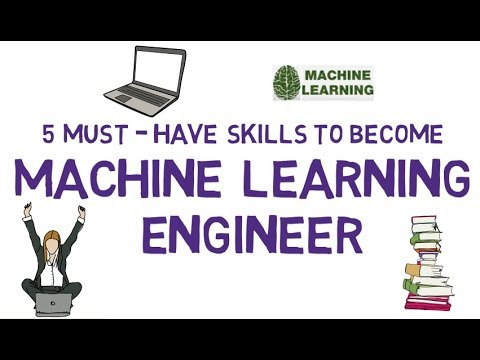# 5 Must Have Skills To Become Machine Learning EngineerLet's check out what are the 5 must-have skills to become a machine learning engineer.

Let's check out what are the 5 must-have skills to become a machine learning engineer.

First, let's understand what machine learning is. In simple words. Machine learning is all about making the computers to perform intelligent tasks without explicitly coding. This is achieved by training the computer with lots and lots of data.

For example: Detecting whether a mail is a spam or not, recognizing handwritten digits, Fraud detection in Transactions... and many such applications...

Now let's see what are the top 5 skills to get a machine learning job.

1). At number 1, we have Math Skills: Under math skills, we need to know probability and statistics, linear algebra and calculus.

Probability and Statistics: Machine learning is very much closely related to statistics.
You need to know the fundamentals of statistics and probability theory, descriptive statistics, Baye's rule and random variables, probability distributions, sampling, hypothesis testing, regression and decision analysis.

Linear Algebra: You need to know how to with matrices and some basic operations on matrices such as matrix addition, subtraction, scalar and vector multiplication, inverse, transpose and vector spaces.

Calculus: In calculus, you need to know the basics of differential and integral calculus.

2). At number two we have Programming skills: A little bit of coding skills is enough. But it's preferred to have the knowledge of data structures, algorithms and Object Oriented Programming (or OOPs) concepts.

Some of the popular programming languages to learn for machine learning is Python, R, Java, and C++.

It's your preference to master any one programming language. But its advisable to have a little understanding of other languages and what their advantages and disadvantages are over your preferred one.

3). At number 3 we have Data engineer skills: Ability to work with large amounts of data (or big data), Data preprocessing, the knowledge of SQL and NoSQL, ETL (or Extract Transform and Load) operations, data analysis and visualization skills.

4). Next, we have Knowledge of Machine Learning Algorithms: you should be familiar with popular machine learning algorithms such as linear regression, logistic regression, decision trees, random forest, clustering (like K means, hierarchical), reinforcement learning and neural networks.

5). And Finally, The Knowledge of Machine Learning Frameworks: You Should be Familiar with popular machine learning frameworks such as sci-kit learn, tensorflow, Azure, caffe, theano, spark and torch.

## How To Build A Data Science Career In 2021

In Conversation With Dr Suman Sanyal, NIIT University,he shares his insights on how universities can contribute to this highly promising sector and what aspirants can do to build a successful data science career.

## Hire Machine Learning Engineer | Offshore Machine Learning Experts

We are a Machine Learning Services provider offering custom AI solutions, Machine Learning as a service & deep learning solutions. Hire Machine Learning experts & build AI Chatbots, Neural networks, etc. 16+ yrs & 2500+ clients.

## The Difference between Data Science, Machine Learning and Big Data!

Many professionals and 'Data' enthusiasts often ask, “What's the difference between Data Science, Machine Learning and Big Data?”. Let's clear the air. If you are still wondering about it then this article is for you.

## 5 stages of learning Data Science

5 stages of learning Data Science and how to ace each of them

## Most popular Data Science and Machine Learning courses — July 2020

Most popular Data Science and Machine Learning courses — August 2020. This list was last updated in August 2020 — and will be updated regularly so as to keep it relevant